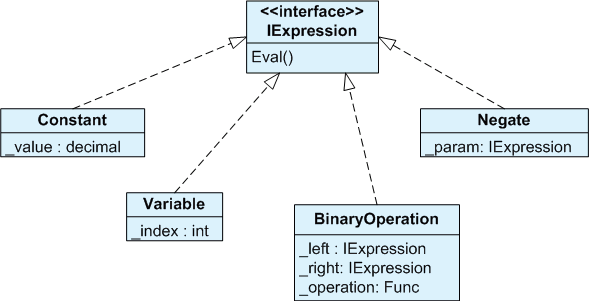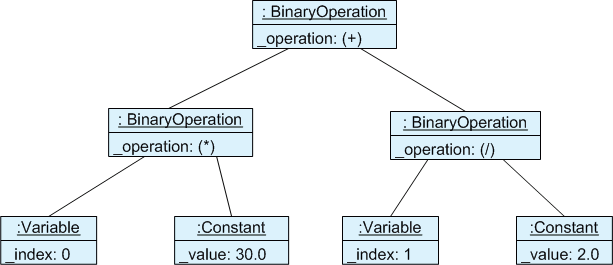# MathConverter: How to Do Math in XAML

## Overview

`MathConverter` is an arithmetic converter for WPF/Silverlight. It allows you to do things like this:

```<RotateTransform Angle="{Binding Text, ElementName=Seconds, Converter={ikriv:MathConverter}, ConverterParameter=x*6}" />
```

or even

```<RotateTransform>
<RotateTransform.Angle>
<MultiBinding Converter="{ikriv:MathConverter}" ConverterParameter="x*30 + y/2">
<Binding Path="Hours" />
<Binding Path="Minutes" />
</MultiBinding>
</RotateTransform.Angle>
</RotateTransform>
```

In other words, it can perform simple calculations directly in XAML eliminating necessity to clutter your view model or write one-off value converters.

Download `MathConverter.cs` (12K C# source code file)

Download demo project (62K ZIP file). Requires Visual Studio 2010, WPF/.NET 3.5, Silverlight 4.

## Background

When working with my XAML files, periodically I run into a need of doing some simple calculations directly in XAML. For instance, what if I want this width to be exactly to thirds of that width, or what if rotation angle is a certain multiple of a particular value? This problem may be solved in a number of ways. Widths are usually better addressed via margins, paddings, or grids. At other times I put calculations into a view model. At some other times I create a special binding converter that would do the arithmetic fo me.

## Why another converter?

Naturally, I am not facing this problem alone. The question of how to do math in XAML has been asked (http://stackoverflow.com/questions/2896989/how-use-math-operations-in-xaml) and answered (http://blogs.msdn.com/b/llobo/archive/2006/11/13/arithmetic-operations-in-xaml.aspx).

Lester Lobo in his blog provides not one, but two converters: his own `ArithmeticConverter` and `JScriptConverter` by Douglas Stockwell. Both of them will do the job under certain circumstances, but they are not perfect. `ArithmeticConverter` is too simple. It can perform only one mathematical operation, and can take only one argument. `JScriptConverter` on the other hand is too powerful. It is pretty much Turing Complete, since you have the whole JScript language in your disposal, but involves compiling dynamic assemblies, and it is not for Silverlight. Compiling dynamic assemblies is slow, it clutters user's disk and may have security implications. To be honest, it seems like an overkill for the most cases of math in XAML.

Generally, you don't want to start actually programming in XAML. Once you have these smart programmable converters it may be tempting to start writing loops and conditions, access files, et cetera et cetera, the sky is the limit. It is not always a good idea. XAML was designed for describing visual representation of GUI widgets. As a programming language it probably would not shine: we have much better tools that allow us debugging, reuse, access control et cetera.

Teleric has a class named Telerik.Windows.Controls.Carousel.ArithmeticValueConverter. Unfortunately, the documentation for this class is minimal. Judging by the samples I could find on the Internet, it is even more limited than Lester's converter. It accepts a single value as a parameter, and probably adds it to the argument (or multiplies argument by it, I am not sure).

All this prompted me to create my own converter, that can perform arbitrary arithmetic, can work in Silverlight, and does not involve dynamic compilation. Yes, it meant I had to write my own parser :) Oh, my college years, it was fun then, it is fun now.

## Using MathConverter

Effectively there are two versions of `MathConverter`: one for WPF and one for Silverlight. They are conditionally compiled from the same source using `#if !SILVERLIGHT` preprocessor directive. The reason for that is two-fold:

• Silverlight does not support multi bindings.
• Silverlight does not support markup extensions.

### Markup Extension Support

Without markup extension, you have to define your converter in a resource (`<ikriv:MathConverter x:Key="name" />`) and then refer to it as `{Binding Converter={StaticResource name} ...}`. With markup extension support you can simply write `{Binding Converter={ikriv:MathConverter} ...}` and this will create a converter instance for you. Markup extension is available only under WPF.

### Supported Expressions

`ConverterParameter` must contain a valid arithmetical expression. Support are the four arithmetic operations, unary plus, unary minus, and parenthesis. Regular priority rules apply: `2+2*2` returns `6`.

For regular bindings the single argument can be referred as `x`, `a`, or `{0}`. These symbols can be used interchangeably. It is not required that the argument appears in the expression. If it does not, the converter will always return the same value. Examples of single argument expressions:

• `42.8`
• `2+2*2`
• `a+1` same as `x+1` same as `{0}+1`
• `(x-1)/(x+1)`
• `-x*(x+9.5)`

For multi-bindings, the first argument may be referred as `a`, `x`, or `{0}`. The second argument is `b`, `y`, or ` {1}`. The third argument is one of `c`, `z`, or `{2}`, the fourth - `d`, `t`, `{3}`. The fifth and further arguments may be referred only by the numeric form `{n}`, where `n` is zero-based argument number. Silverlight does not support multi-bindings. Examples of multi-binding expression:

• `42.8`
• `a+b*c`
• `(x+y)/(z-1)`
• `{0}+2*{1}+3*{2}*2.7818*{3}+3.1416*{4}`

All calculations are performed in `decimal`. The result is then converted to the target type, that can be `string`, `int`, `double`, `long`, or `decimal`. In case the calculation resulted in an error (malformed expression, overflow, division by zero, unknown target type) the return value is `DependencyPropety.UnsetValue` and the exception text is written to the Visual Studio output window, e.g. "`MathConverter: error parsing expression 'x*6+'. Unexpected end of text at position 4"`.

## Sample Project

The sample project contains the converter, a WPF sample, a Silverlight sample and unit tests. The Silverlight sample application is demonstrated below.

The demo project contains the `MathConverter`Sample silverlight application is demonstrated below. The rotation angle of the clock hands is calculated in XAML using `MathConverter`.

### Silverlight

```<!-- Silverlight -->
<UserControl ...>
<UserControl.Resources>
<ikriv:MathConverter x:Key="MathConverter" />
</UserControl.Resources>
<Grid ...>
<TextBox Name="Hours" ... />
<TextBox Name="Minutes" ... />
<TextBox Name="Seconds" ... />

<!-- small hand (hours) -->
<Line X1="0" Y1="0" X2="0" Y2="-35" Stroke="Black" StrokeThickness="4">
<Line.RenderTransform>
<RotateTransform Angle="{Binding Text, ElementName=Hours,Converter={StaticResource MathConverter}, ConverterParameter=x*30}" />
</Line.RenderTransform>
</Line>

<!-- big hand (minutes) -->
<Line X1="0" Y1="0" X2="0" Y2="-40" Stroke="Black" StrokeThickness="3">
<Line.RenderTransform>
<RotateTransform Angle="{Binding Text, ElementName=Minutes,Converter={StaticResource MathConverter}, ConverterParameter=x*6}" />
</Line.RenderTransform>
</Line>

<!-- seconds hand -->
<Line X1="0" Y1="0" X2="0" Y2="-40" Stroke="Black" StrokeThickness="1">
<Line.RenderTransform>
<RotateTransform Angle="{Binding Text, ElementName=Seconds,Converter={StaticResource MathConverter}, ConverterParameter=x*6}" />
</Line.RenderTransform>
</Line>
</Grid>
</UserControl>
```

### WPF

Silverlight does not support multi-bindings, so the position of the hour hand depends only on the whole hours, but not on the minutes. I.e. at 10:59:59 it would still point to 10 o'clock sharp. We can do better in the WPF version by combining hours and minutes, so at 10:59:59 the hour hand points almost to 11 o'clock:

```<!-- WPF -->

<!-- small hand (hours) -->
<Line X1="0" Y1="0" X2="0" Y2="-35" Stroke="Black" StrokeThickness="4">
<Line.RenderTransform>
<RotateTransform>
<RotateTransform.Angle>
<MultiBinding Converter="{ikriv:MathConverter}" ConverterParameter="x*30 + y/2">
<Binding Path="Text" ElementName="Hours" />
<Binding Path="Text" ElementName="Minutes" />
</MultiBinding>
</RotateTransform.Angle>
</RotateTransform>
</Line.RenderTransform>
</Line>```

## Inside the Converter's Code

### The Parser

The heart of the converter is the parser. Its job is to parse the expression text such as `x*30 + y/2` and convert it to an object of type `IExpression`, which represents the calculation tree (see below). Thus, the parser's public interface has only one method:

```class Parser
{
public IExpression Parse(string text);
}```
`Parser` is a classic recursive descent parser It uses simple arithmetical expressions grammar, a variant of which can be found in virtually every book on compilers (e.g. Aho et al., p. 77):.

```Expression ::= Expression + Term
Term ::= Term * Factor
Term ::= Term / Factor
Factor ::= constant
Factor ::= variable
Factor ::= -Factor
Factor ::= +Factor
Factor ::= (Expression)```

Each non-terminal symbol of the grammar is handled by a corresponding parsing method: `Parser.ParseExpression()`, `Parser.ParseTerm()`, `Parser.ParseFactor()`. The parser also acts as a primitive lexer by skipping whitespaces and parsing decimal numbers using a RegEx.

### Expression Trees

The parser produces a single object that implements interface `IExpression`, which is defined as folows:

```interface IExpression
{
decimal Eval(object[] args);
}```

The array passed to the `Eval` method contains concrete values of the variables involvedin the expression. E.g. for expression `x*30 + y/2` we expect to find value of `x` in `args` and value of `y` in `args`. For a regular binding there will be only one argument that is a value of the source property. For a multibinding there may be multiple arguments which are the values of the source properties.

There are several concrete implementations of the `IExpression` interface:• `Constant.Eval(args)` always returns the same value, e.g. `30.0`
• `Variable.Eval(args)` returns `args[index]` where `index` is the variable number (x=0, y=1, etc.)
• `Negate` class holds another expression. `Negate.Eval()` evaluates that experssions and negates the result.
• `BinaryOperation` holds two experssions and an operation function (multiplication, division, addition, etc.). `BinaryOperation.Eval()` evaluates inner experssions and applies the function to the results.

For the expression text `x*30+y/2` the parser would generate the following expression tree:After that all the converter needs to do is to call `Eval()` on the top expression and supply the arguments.

### Type Safety

The converter performs all calcualtions using type `decimal`. Literal constants are converted to `decimal` using `decimal.TryParse()`. Variable (source propety) values are converted using `System.Convert.ToDecimal()`. The output value can then be converted to `decimal`, `string`, `long`, `int`, or `double`. Other target types are not supported.

### Markup Extension

Under WPF, the converter derives from `MarkupExtension` class, that allows to create instances of the converter in XAML using `{ikriv:MathConverter}` syntax, where `ikriv` is XAML namespace mapped to CLR namespace `Ikriv.Wpf`. Silverlight does not support markup extensions as of now, so you'll need to define an instance of the converter in your resources.

### Conclusion

I tried to make the code as self-explanatory and simple as possible. There are not too many comments, but the methods are short and the names are descriptive. The expression grammar I am parsing is very small, if not trivial. You don't have to know the theory of parsing in order to understand how the parser works. One may want to extend the grammar to support function calls, array argumetns and the like, but this was way beyond the scope of what I needed. I hope you enjoy this converter and find it valuable.